# GCF and LCM

Here you will learn about GCF and LCM (greatest common factor and least common multiple), including how to find the GCF and LCM of two or more numbers using the prime factorization method and recognize when to find the GCF or the LCM in word problems.

Students will first learn about GCF and LCM as part of the number system in 6th grade.

## What is GCF and LCM?

GCF and LCM are two abbreviations for the greatest common factor (GCF) and the least common multiple (LCM).

• The GCF is the largest whole number that two or more numbers can be divided by. Other names for this include the greatest common divisor (GCD) and the highest common factor (HCF).

For example, find the GCF of 8 and 12.

Let’s start by writing the factors of 8 and 12.

Factors of {\bf{8}} {\textbf{: }} 1, 2, 4, 8
Factors of {\bf{12}} {\textbf{: }} 1, 2, 3, 4, 6, 12

There are several numbers that occur in both lists ( 1, 2, and 4 ).

The largest factor that occurs in each list is 4, and so the greatest common factor of 8 and 12 is \bf{4}.

• The LCM is the smallest whole number that is a multiple of two or more whole numbers (exists within the multiplication table of each number). Another name for this is the lowest common multiple.

For example, find the LCM of 8 and 12.

Let’s start by writing the first 12 multiples of 8 and 12.

Multiples of {\bf{8}} {\textbf{: }} 8, 16, 24, 32, 40, 48, 56, 64, 72, 80, 88, 96
Multiples of {\bf{12}} {\textbf{: }} 12, 24, 36, 48, 60, 72, 84, 96, 108, 120, 132, 144

There are several values that occur in both lists ( 24, 48, 72, and 96 ). The lowest of these is 24, hence the least common multiple of 8 and 12 is \bf{24}.

## Prime factor decomposition

To calculate the GCF or LCM of two or more numbers, you can write out a list of factors or multiples as we have above, however, this approach can be very time consuming and can be complicated when dealing with factors and multiples of large numbers ( 3 digit numbers in particular).

You can therefore use prime factorization to find these values.

The fundamental theorem of arithmetic states that every positive whole number greater than one is either a prime number, or can be written as a product of its prime factors. Every number has a unique set of numbers called prime factors.

By presenting prime factors within a Venn diagram, you can quickly determine both the GCF and LCM of the two or more numbers in the question.

For example,

8=2\times{2}\times{2}

12=2\times{2}\times{3}

The intersection of the two circles contains the greatest common factor, where you multiply the values within the intersection together.

Here, the GCF of 8 and 12 is equal to 2\times{2}=4.

The union of the two circles contains the least common multiple where you multiply the values within both circles together.

Here, the LCM of 8 and 12 is equal to 2\times(2\times{2})\times{3}=24.

As the least common multiple is found by multiplying all of the factors together within the Venn diagram, the least common multiple can be found by multiplying the greatest common factor by the remaining prime factors.

\text{LCM } = \text{ GCF } \times \text{ Remaining prime factors}

This allows you to solve problems where you are given the GCF and LCM of two numbers and you need to determine the original two numbers.

### What is GCF and LCM?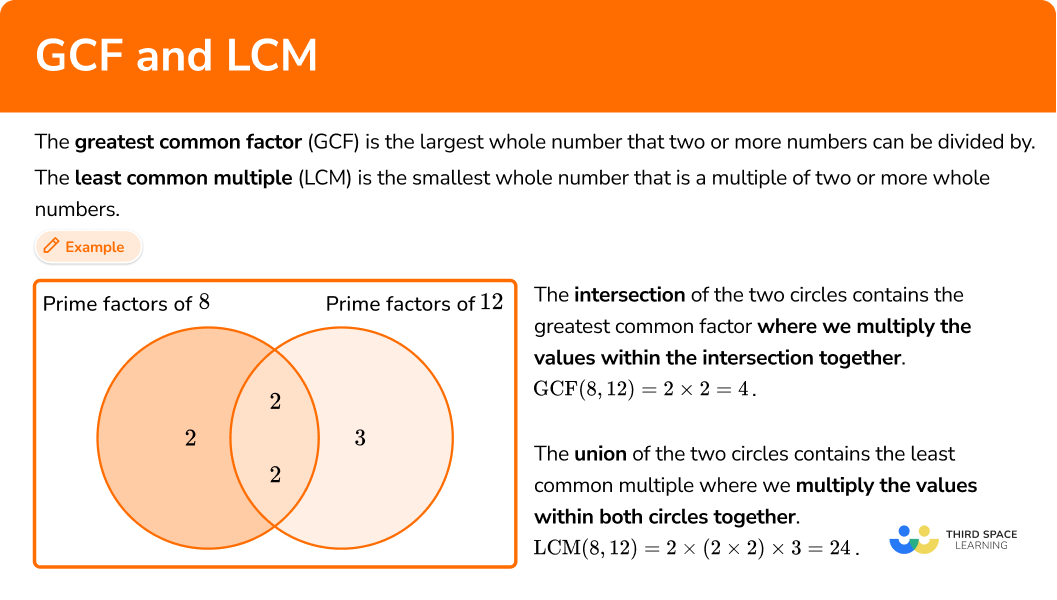## Common Core State Standards

How does this relate to 6th grade math?

• Grade 6 – The Number System (6.NS.4)
Find the greatest common factor of two whole numbers less than or equal to 100 and the least common multiple of two whole numbers less than or equal to 12. Use the distributive property to express a sum of two whole numbers (1–100) with a common factor as a multiple of a sum of two whole numbers with no common factor. For example, express 36 + 8 \, as \, 4 (9 + 2).

## How to find the greatest common factor

In order to find the greatest common factor of two or more numbers:

1. State the product of prime factors for each number.
2. Write all the prime factors for each number into a Venn diagram.
3. Multiply the prime factors in the intersection to find the GCF.

## How to find the least common multiple

In order to find the least common multiple of two or more numbers:

1. State the product of prime factors for each number.
2. Write all the prime factors for each number into a Venn diagram.
3. Multiply each prime factor in the Venn diagram to find the LCM.

## GCF and LCM examples

### Example 1: GCF of two simple composite numbers

Find the greatest common factor of 30 and 42.

1. State the product of prime factors for each number.

30=2\times{3}\times{5}

42=2\times{3}\times{7}

2Write all the prime factors for each number into a Venn diagram.

3Multiply the prime factors in the intersection to find the GCF.

GCF =2\times{3}=6.

### Example 2: LCM of two simple composite numbers

Calculate the least common multiple of 16 and 18.

State the product of prime factors for each number.

Write all the prime factors for each number into a Venn diagram.

Multiply each prime factor in the Venn diagram to find the LCM.

### Example 3: GCF word problem

120 \ ml of red paint and 156 \ ml of blue paint are mixed together to create a tin of purple paint. The paint is then distributed equally into sample tubes. Each tube must contain the same amount of paint that must be over 20 \ ml.

What is the maximum number of tubes that can be filled with the minimum amount of paint?

State the product of prime factors for each number.

Write all the prime factors for each number into a Venn diagram.

Multiply the prime factors in the intersection to find the GCF.

### Example 4: LCM word problem

A plumber is fixing multiple leaking pipes. Pipe A drips water every 12 seconds. Pipe B drips water every 22 seconds. Both pipes drip at the same time. How much time passes before they next drip at the same time? Write your answer using minutes and seconds.

State the product of prime factors for each number.

Write all the prime factors for each number into a Venn diagram.

Multiply each prime factor in the Venn diagram to find the LCM.

## How to find the original values given the GCF and the LCM

In order to find the original values given the GCF and the LCM:

1. Divide the LCM by the GCF.
2. Calculate the product of primes of the remainder.
3. Determine which prime factors match each original number.

### Example 5: find the numbers, given the GCF

The greatest common factor of 3 numbers is 7. The product of their remaining prime factors is 30 and each number is greater than 10. Determine the value of the three numbers.

Divide the LCM by the GCF to determine the remainder.

Calculate the product of primes of the remainder.

Determine which prime factors match each original number.

### Example 6: find the original numbers given the GCF and LCM

Two numbers, A and B, have the following number properties:

• GCF (A,B) = 7
• LCM (A,B) = 2,310
• A is divisible by 3
• B is an even number
• 100<A<B

Determine the values of A and B.

Divide the LCM by the GCF to determine the remainder.

Calculate the product of primes of the remainder.

Determine which prime factors match each original number.

### Tips for teaching GCF and LCM

• Before introducing GCF and LCM, students should have a strong understanding of factors and multiples, which they would have first learned in 4th grade. Review these terms and practice listing factors and multiples if needed.

### Easy mistakes to make

• Finding the GCF instead of the LCM (and vice versa)
A very common misconception is mixing up the greatest common factor with the least common multiple. Factors are composite numbers that are split into smaller factors. Multiples are composite numbers that are multiplied to make larger numbers.

• Incorrect evaluation of powers
It is possible to write prime factors into a Venn diagram with their associated exponent or power. This only becomes an issue when the powers are not correctly interpreted. We suggest having students write the prime factors without the use of exponents.
Take, for example, the numbers 12 and 18.
12=2^{2}\times{3}
18=2\times{3}^{2}
Here, 2^{2}=2\times{2}=4 which is correct, however, the same misconception could then be continued to 3^{2}=3\times{2}=6, which is incorrect. Instead, 3^{2}=3\times{3}=9. This will have an impact on the value of the GCF and the LCM.

### Practice GCF and LCM questions

1. Find the GCF of 54 and 60.

540366254=2\times{3}\times{3}\times{3}

60=2\times{2}\times{3}\times{5}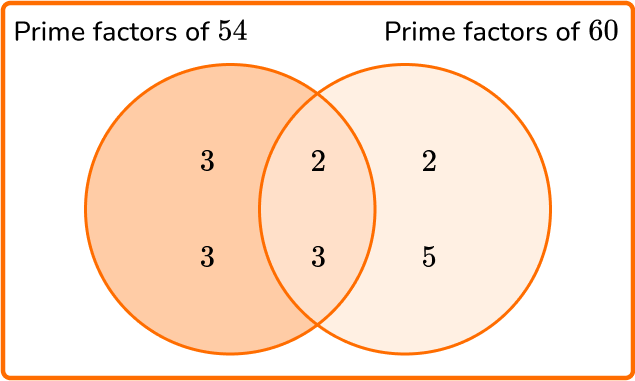GCF (54,60) = 2\times{3}=6

2. Find the LCM of 24 and 32.

8169676824=2\times{2}\times{2}\times{3}

32=2\times{2}\times{2}\times{2}\times{2}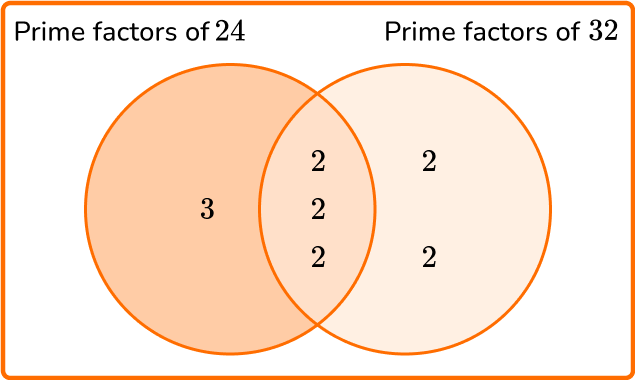LCM (24,32) = 3\times(2\times{2}\times{2})\times(2\times{2})=3\times{8}\times{4}=96

3. Two lengths of ribbon measure 1.2 \ m and 80 \ cm. Each piece of ribbon needs to be cut into the fewest number of pieces of the same length. What is the length of each piece?

12 \ cm40 \ cm0.1 \ m240 \ cm1.2 \ m = 120 \ cm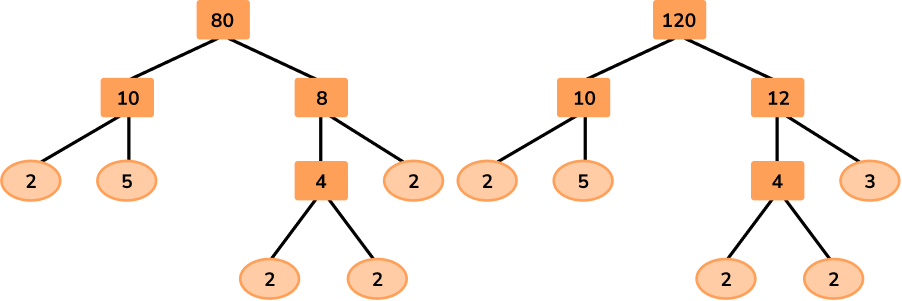80=2\times{2}\times{2}\times{2}\times{5}

120=2\times{2}\times{2}\times{3}\times{5}

GCF (80,120) = 2\times{2}\times{2}\times{5}=40

4. Two runners leave the start line of a 200 \ m track on the whistle. It takes runner A \ 1 minute to run 1 lap of the track and runner B \ 1 minute and 12 seconds. What distance will runner B have traveled when they next cross the start line at the same time?

1.2 \ km1 \ km800 \ m200 \ mConverting both lap times to seconds, runner A takes 60 seconds, and runner B takes 72 seconds.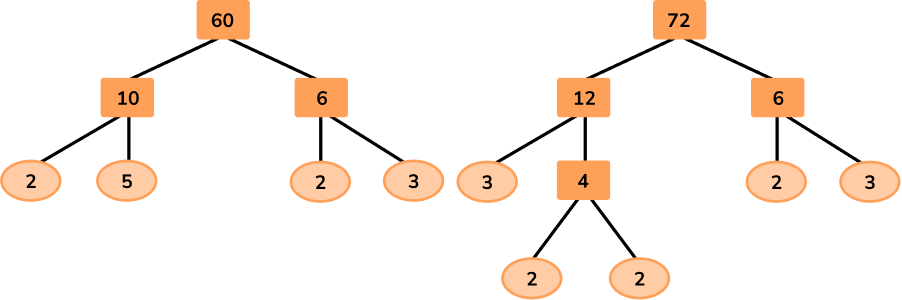60=2\times{2}\times{3}\times{5}

72=2\times{2}\times{2}\times{3}\times{3}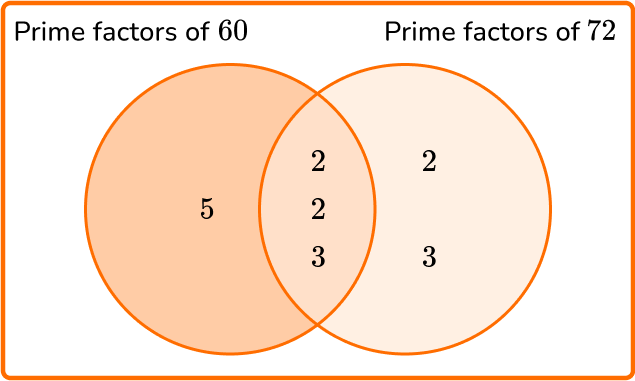GCF (60,72)=2\times{2}\times{3}=12

LCM (60,72) = 5 \times 12 \times (2 \times 3)=5 \times 12 \times 6=360

360 seconds = 6 minutes

6\div{1.2}=5 laps

5\times{200}=1000 \ m=1 \ km

5. The greatest common factor of two numbers is 35. The product of the remaining factors is 33. Both original numbers contain three digits. What is the difference between the two original numbers?

2112028038533=3\times{11}

Smaller number: 35\times{3}=105

Larger number: 35\times{11}=385

385-105 = 280

6. Two numbers x and y have the following number properties:

• \text{LCM }(x,y)=96
• \text{GCF }(x,y)=8
• 2<x<y<40

What is the value of x+y?

56641042396\div{8}=12

12=2\times{2}\times{3}

8\times{2}\times{2}=32

8\times{3}=24

32+24=56

## GCF and LCM questions

1. A farm needs to divide their two fields into equal-sized enclosures for some horses. Field 1 is 240 \ m^2. Field 2 is 160 \ m^2. Each horse must have at least 42 \ m^2.

(a) What is the minimum possible area for each enclosure?

(b) What is the maximum number of horses that can use these two fields?

(a)

240=2^4 \times 3 \times 5 \, or \, 240=2 \times 2 \times 2 \times 2 \times 3 \times 5

160=2^5 \times 5 \, or \, 160=2 \times 2 \times 2 \times 2 \times 2 \times 5

GCF (240,160)=80 \ m^2

(b)

2+3=5 \, or \, (240+160) \div 80=5

2. Given that 6480=2^4 \times 3^4 \times 5, simplify the ratio 10800:6480.

10800=2^4 \times 3^3 \times 5^2

GCF (10800,6480)=2^4 \times 3^3 \times 5

Remaining factors are 5 (for 10800 ) and 3 (for 6480 ).

5:3

3. The least common multiple of x and y is 2^3 \times 3^2 \times 5^2 where x is a square number such that 36<x<225.

(a) Find the exact value of x.

(b) The greatest common factor of x and y is 4. Determine the value of y. Use the Venn diagram below to help you.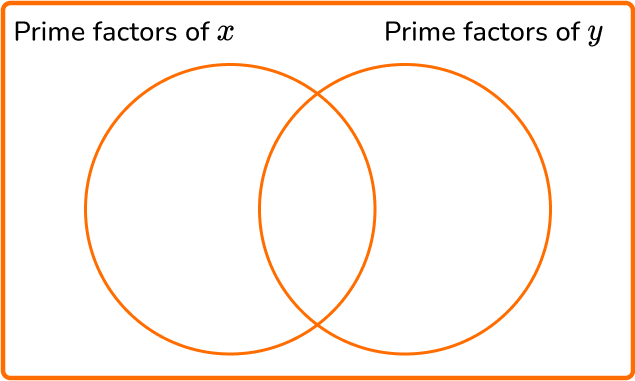(a)

x=2^2 \times 5^2 or

x=100

(b)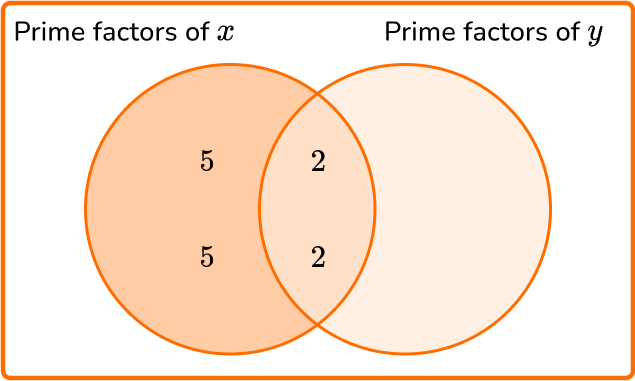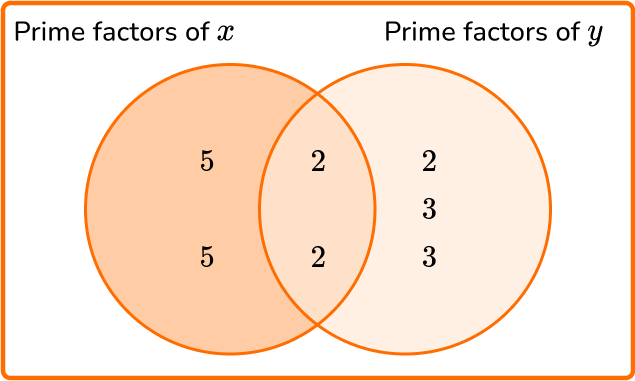y=(2 \times 2) \times (2 \times 3 \times 3)=4 \times 18=72

## GCF and LCM FAQs

How do you find the GCF?

Step 1: State the product of prime factors for each number.
Step 2: Write all the prime factors for each number into a Venn diagram.
Step 3: Multiply the prime factors in the intersection to find the GCF.

How do you find the LCM?

Step 1: State the product of prime factors for each number.
Step 2: Write all the prime factors for each number into a Venn diagram.
Step 3: Multiply each prime factor in the Venn diagram to find the LCM.

What is the prime factorization method of finding the GCF & LCM?

To find the GCF, list all prime factors that are common between the two numbers and multiply them together. To find the LCM, multiply the GCF by all the prime factors of both numbers that have not yet been used.

What is the difference between least common multiple (LCM) and least common denominator (LCD)?

The least common denominator (LCD) is the least common multiple (LCM) of the denominators of two or more fractions.

## Still stuck?

At Third Space Learning, we specialize in helping teachers and school leaders to provide personalized math support for more of their students through high-quality, online one-on-one math tutoring delivered by subject experts.

Each week, our tutors support thousands of students who are at risk of not meeting their grade-level expectations, and help accelerate their progress and boost their confidence.